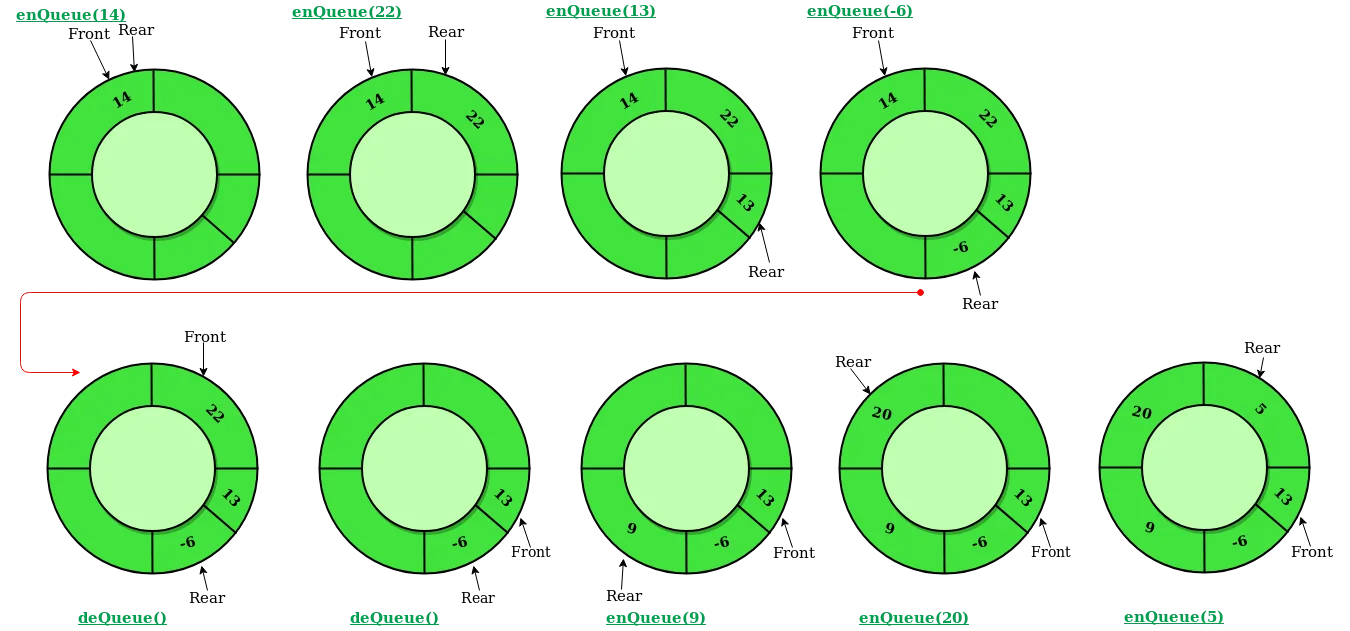## 队列#

public class Node
{
public int data;
public Node next;

public Node(int data)
{
this.data = data;
}
}

{
public Node tail;

// 插入一个元素
public void Enqueue(int data)
{
Node newNode = new Node(data);
if (head == null)
{
tail = newNode;
}
else
{
tail.next = newNode;
tail = newNode;
}
}

// 删除一个元素
public int Dequeue()
{
if (IsEmpty())
{
Debug.LogError("Is Empty");
return -1;
}

Node node = head;
return node.data;
}

// 判断是否为空
public bool IsEmpty()
{
return head == null;
}
}


## 循环队列#public class CircularQueue
{
private int[] array = null;
private int capacity = 0;

public int head = 0;
public int tail = 0;

// 在New一个循环队列时，指定队列的容量
public CircularQueue(int capacity)
{
this.capacity = capacity;
this.array = new int[capacity];
}

// 判断队列是否为空
public bool IsEmpty()
{
return head == tail;
}

// 判断队列是否已满
public bool IsFull()
{
return (tail + 1) % capacity == head;
}

// 入列一个元素
public void Enqueue(int data)
{
if (IsFull())
{
Debug.LogError("Queue is Full!");
return;
}
array[tail] = data;
tail = (tail + 1) % capacity;
Debug.Log("Enqueue: " + data);
}

// 出列一个元素
public int Dequeue()
{
if (IsEmpty())
{
Debug.LogError("Queue Is Empty");
return -1;
}

int data = array[head];
head = (head + 1) % capacity;
return data;
}
}


## 双端队列#

public class DoubleEndedQueue
{
public Node left = null;
public Node right = null;

// 判断队列是否为空
public bool IsEmpty()
{
return (left == null || right == null);
}

// 从左边入列一个元素
public void EnqueueLeft(int data)
{
Node newNode = new Node(data);
if(left == null)
{
left = newNode;
right = newNode;
}
else
{
newNode.next = left;
left.prev = newNode;
left = newNode;
}
}

// 从左边出列一个元素
public int DequeueLeft()
{
if (IsEmpty())
{
return -1;
}

Node node = left;

if(left.next != null)
{
left.next.prev = null;
}

left = left.next;

return node.data;
}

// 从右边入列一个元素
public void EnqueueRight(int data)
{
Node newNode = new Node(data);
if(right == null)
{
left = newNode;
right = newNode;
}
else
{
newNode.prev = right;
right.next = newNode;
right = newNode;
}
}

// 从右边出列一个元素
public int DequeueRight()
{
if (IsEmpty())
{
return -1;
}

Node node = right;
if(right.prev != null)
{
right.prev.next = null;
}

right = right.prev;

return node.data;
}
}